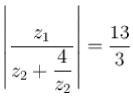Courses

# Complex Number MSQ

## 10 Questions MCQ Test Topic wise Tests for IIT JAM Physics | Complex Number MSQ

Description
This mock test of Complex Number MSQ for Physics helps you for every Physics entrance exam. This contains 10 Multiple Choice Questions for Physics Complex Number MSQ (mcq) to study with solutions a complete question bank. The solved questions answers in this Complex Number MSQ quiz give you a good mix of easy questions and tough questions. Physics students definitely take this Complex Number MSQ exercise for a better result in the exam. You can find other Complex Number MSQ extra questions, long questions & short questions for Physics on EduRev as well by searching above.
*Multiple options can be correct
QUESTION: 1

### If n is the smallest positive integer for which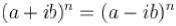where a > 0 and b > 0 then the numerical value of b/a is :

Solution:

n = 1 ⇒ b = 0 not possible
n = 2 ⇒ a = 0 or b = 0 not possible
n = 3 ⇒ a3 - ib3 + 3a2 bi - 3ab2 = a3 + ib3 -3a2 bi - 3ab2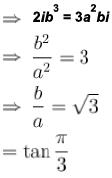The correct answers are: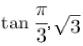*Multiple options can be correct
QUESTION: 2

### If α,β be the roots o f the equation μ2 - 2μ + 2 = 0 and if cos θ = x + 1, then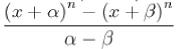is equal to :

Solution: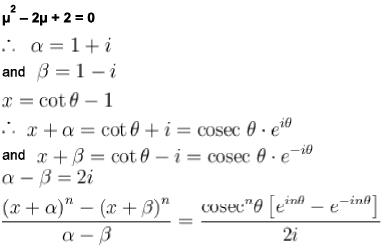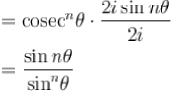The correct answers are: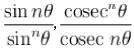*Multiple options can be correct
QUESTION: 3

### The complex numbers associated with the vertices A, B, C of Δ ABC are eiθ, ω,ω2 respectively, where ω is imaginary cube root of unity and cos θ > Re (ω), then the complex number representing the point where angle bisector of ∠ A meets the circumcircle of triangle, is :

Solution: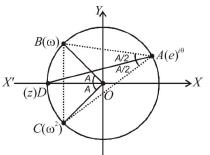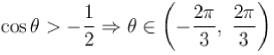⇒ A lies on major are BC∴ angle subtended by are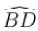at O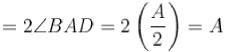The correct answers are: ω + ω2,-1

*Multiple options can be correct
QUESTION: 4

If ω is an imaginary cube root of unity such that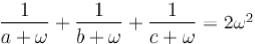and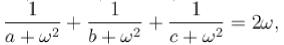then

Solution:

ω and ω are roots of the equation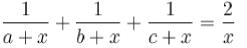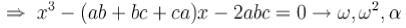sum of roots = ω + ω2 + α = 0
⇒ α = 1
⇒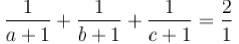The correct answers are: 2, 3, 4

*Multiple options can be correct
QUESTION: 5

Which of the following statement are true.

Solution: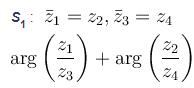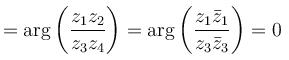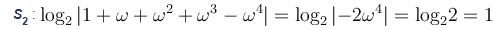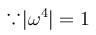S3 : Since z1 z2 are 8th roots of unity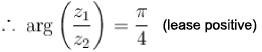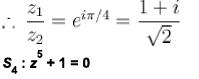∴ product of all the roots = (-1)5 . 1 = -1
The correct answers are: S2 : If ω is an imaginary fifth root of unity, then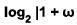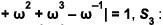If z1 and z2 are two of the 8th roots o f unity, such that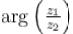is least positive, then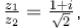S4 : The product of all the fifth roots of-1 is equal to-1

*Multiple options can be correct
QUESTION: 6

If z1 lies on |z| = 1 and  z2  lies on |z| = 2, then :

Solution: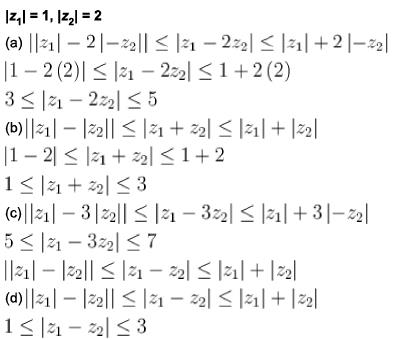The correct answers are: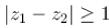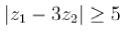,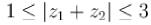,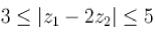*Multiple options can be correct
QUESTION: 7

lf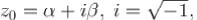then roots of the equation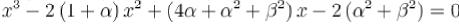are:

Solution: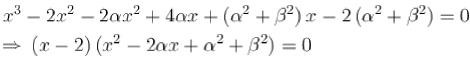The correct answers are: z0,2,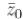*Multiple options can be correct
QUESTION: 8

Let z1,and z2 be non-zero complex numbers satisfying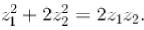Then the triangle made by points with vertices at origin, z1 and z2 is :

Solution: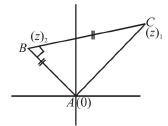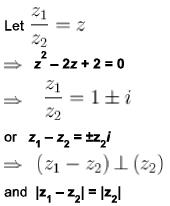The correct answers are: an isosceles triangle, a right angled isosceles triangle

*Multiple options can be correct
QUESTION: 9

If z is a complex number satisfying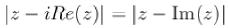then z lies on :

Solution: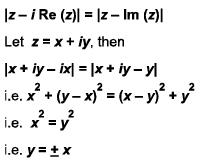The correct answers are: y = x, y = - x

*Multiple options can be correct
QUESTION: 10

If  z1 = 5 + 12i and |z2| = 4 then :

Solution:

z1 5 + 12i,|z2| = 4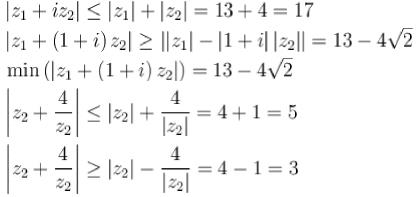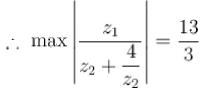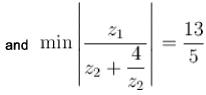The correct answers are: maximum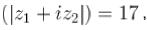maximum